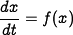# FAQ: Can we get more information about state space formulation?

Jump to: navigation, search

Submitted by: Steve Waydo
Submitted on: October 9, 2003

"State-space" refers to modeling a system using some number of quantities, called the states, that are sufficient to fully charactarize its behavior. For example, the spring-mass-damper system we see so frequently can be fully described by two states: the position and velocity of the mass. We know this is true because when we solve the ODE if we know the position and velocity at a given instant that is all we need to fully charactarize the behavior - no information about past behavior or other quantities is needed. When we model a system using state-space methods, we always end up with a system of first order differential equations in the states,It is important to note that our choice of states is not unique, but the number of states needed to describe a given system is fixed. For example, for a coupled spring mass system where we have two masses and two springs we can use the position and velocity of each mass as our states (4 states), but we can just as well use the sum and difference of the positions of the two masses and their derivatives (also 4 states) and we will get the same answers.